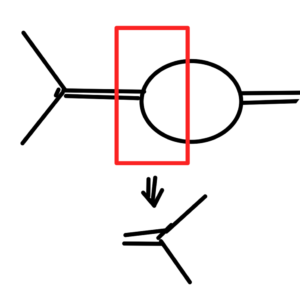I’ve now uploaded a new version of my class notes for PHY2403, the UofT Quantum Field Theory I course, taught this year by Prof. Erich Poppitz.

This version includes the following chapters, roughly one per lecture, plus some extras

• Introduction
• Units, scales, and Lorentz transformations.
• Lorentz transformations and a scalar action.
• Scalar action, least action principle, Euler-Lagrange equations for a field, canonical quantization.
• Klein-Gordon equation, SHOs, momentum space representation, raising and lowering operators.
• Canonical quantization, Simple Harmonic Oscillators, Symmetries
• Symmetries, translation currents, energy momentum tensor.
• 1st Noether theorem, spacetime translation current, energy momentum tensor, dilatation current.
• Unbroken and spontaneously broken symmetries, Higgs Lagrangian, scale invariance, Lorentz invariance, angular momentum quantization
• Lorentz boosts, generator of spacetime translation, Lorentz invariant field representation.
• Microcausality, Lorentz invariant measure, retarded time SHO Green’s function.
• Klein-Gordon Green’s function, Feynman propagator path deformation, Wightman function, Retarded Green’s function.
• Forced Klein-Gordon equation, coherent states, number density, time ordered product, perturbation theory, Heisenberg picture, interaction picture, Dyson’s formula
• Time evolution, Hamiltonian pertubation, ground state
• Perturbation ground state, time evolution operator, time ordered product, interaction
• Differential cross section, scattering, pair production, transition amplitude, decay rate, S-matrix, connected and amputated diagrams, vacuum fluctuation, symmetry coefficient
• Scattering, decay, cross sections in a scalar theory.
• Problem Set 1.
• Problem Set 2.
• Independent study problems
• Useful formulas and review.
• Momentum of scalar field.
• Index
• Bibliography

Problem set 1-2 solutions are redacted.  If you aren’t a UofT student taking PHY2403, feel free to contact me for an un-redacted copy.